# Difference between revisions of "Butane"This article is a 'stub' page, it has no, or next to no, content. It is here at the moment to help form part of the structure of SklogWiki. If you add sufficient material to this article then please remove the {{Stub-general}} template from this page.

  200 lightgrey butane.pdb  n-butane

Butane (C4H10) (n-butane). The NERD parameters are:

 Molecule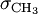$\sigma_{\mathrm {CH}_3}$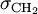$\sigma_{\mathrm {CH}_2}$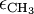$\epsilon_{\mathrm {CH}_3}$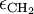$\epsilon_{\mathrm {CH}_2}$ butane 3.91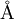$\mathrm{\AA}$ 3.93$\mathrm{\AA}$ 104.0 K 45.8 K

## Isobutane

Isobutane is the branched isomer of butane.

  200 lightgrey isobutane.pdb  Isobutane